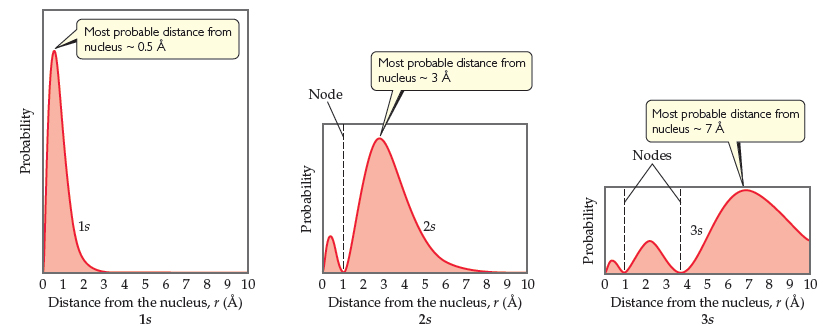# Problem: Radial probability distributions for the 1s , 2s , and 3s  orbitals of hydrogen. These plots show the probability of finding the electron as a function of distance from the nucleus. As n increases, the most likely distance at which to find the electron (the highest peak) moves farther from the nucleus.How many maxima would you expect to find in the radial probability function for the 4s  orbital of the hydrogen atom?

###### FREE Expert Solution

From the radial probability distribution plots, we can see that:

• principal quantum number, n → corresponds to n peaks (or maxima)
• 1s has 1 maxima, 2s has 2 maxima, 3s has 3 maxima###### Problem DetailsRadial probability distributions for the 1s , 2s , and 3s  orbitals of hydrogen. These plots show the probability of finding the electron as a function of distance from the nucleus. As n increases, the most likely distance at which to find the electron (the highest peak) moves farther from the nucleus.

How many maxima would you expect to find in the radial probability function for the 4s  orbital of the hydrogen atom?